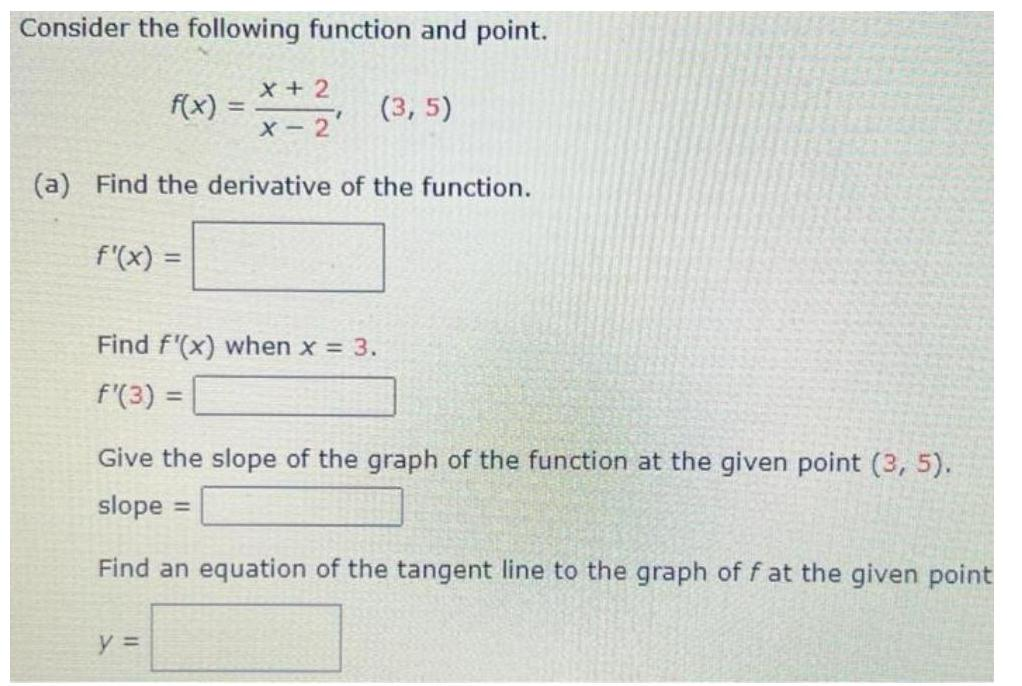Question:

# Consider the following function and point. F(x) = (x +

Last updated: 7/7/2022Consider the following function and point. F(x) = (x + 2)/(x-2)₁ (3,5) (a) Find the derivative of the function. f'(x) = Find f'(x) when x = 3. f'(3) = Give the slope of the graph of the function at the given point (3, 5). slope Find an equation of the tangent line to the graph of f at the given point y =